• Slides: 36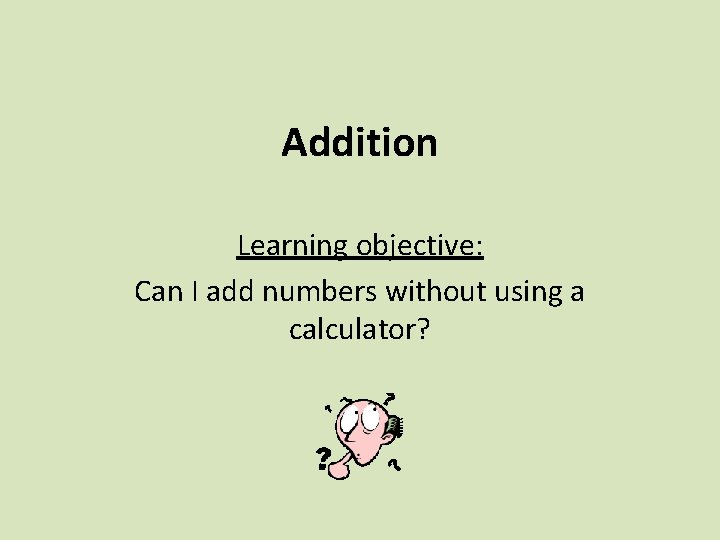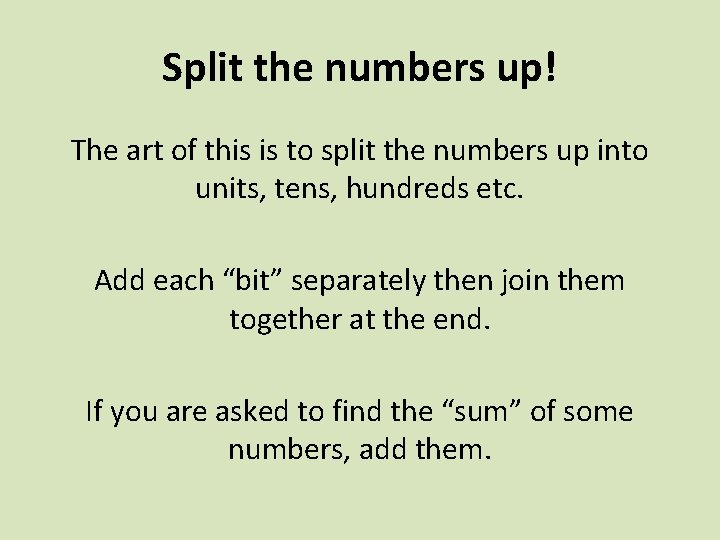Split the numbers up! The art of this is to split the numbers up into units, tens, hundreds etc. Add each “bit” separately then join them together at the end. If you are asked to find the “sum” of some numbers, add them.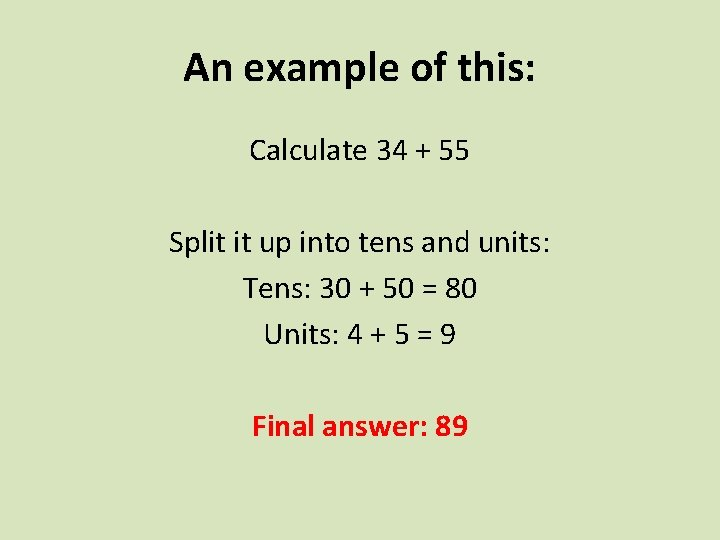An example of this: Calculate 34 + 55 Split it up into tens and units: Tens: 30 + 50 = 80 Units: 4 + 5 = 9 Final answer: 89Another example of this: Calculate 187 + 264 Split it up into tens and units: Hundreds: 100 + 200 = 300 Tens: 80 + 60 = 140 Units: 7 + 4 = 11 Final answer: 451Try these: 1. 26 + 53 = 79 2. 57 + 35 = 92 3. 98 + 57 = 155 4. 365 + 478 = 843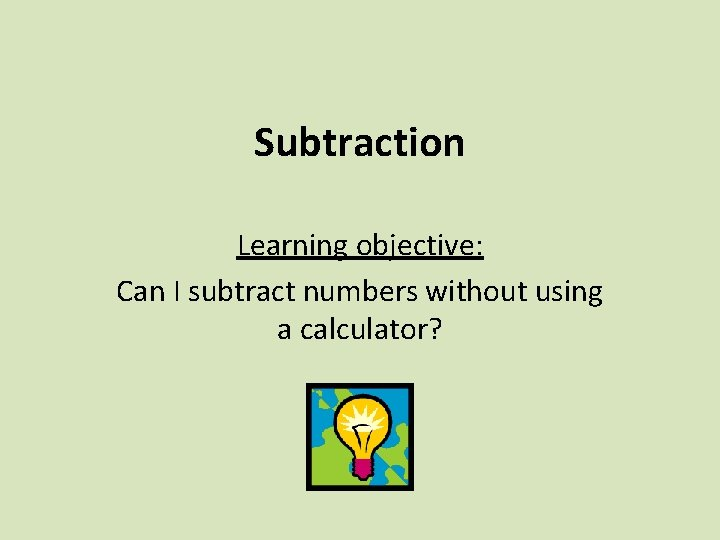Subtraction Learning objective: Can I subtract numbers without using a calculator?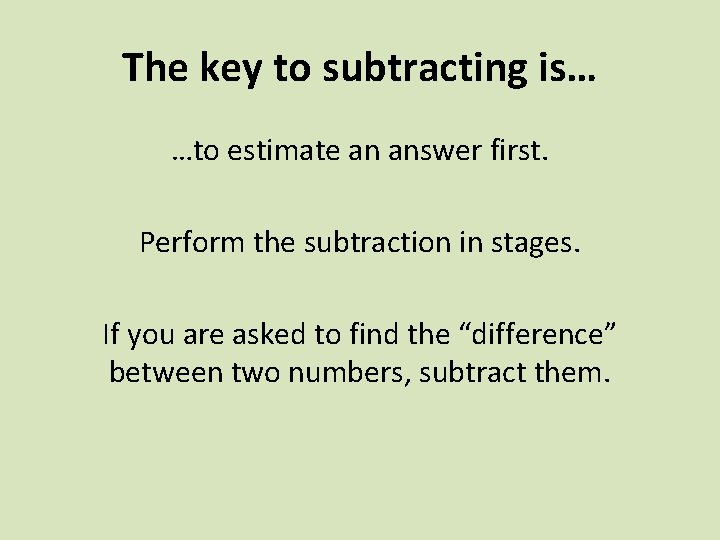The key to subtracting is… …to estimate an answer first. Perform the subtraction in stages. If you are asked to find the “difference” between two numbers, subtract them.What does this mean? Calculate 72 – 38 Make it easier: 72 – 30 Answer: 42 42 - 8 Answer: 34 Firstly take subtract 30 Then subtract 8A different method: Calculate 72 – 38 Make it easier: 72 – 40 Answer: 32 Actual answer: 34 +2 to the number you’re subtracting +2 to your answerAnother example: Calculate 91 – 23 Make it easier: 91 – 20 Answer: 71 71 - 3 Actual answer: 68 Subtract 20 Subtract 3Try these: 1. 85 - 36 = 49 2. 52 - 25 = 27 3. 96 - 58 = 38 4. 124 - 89 = 35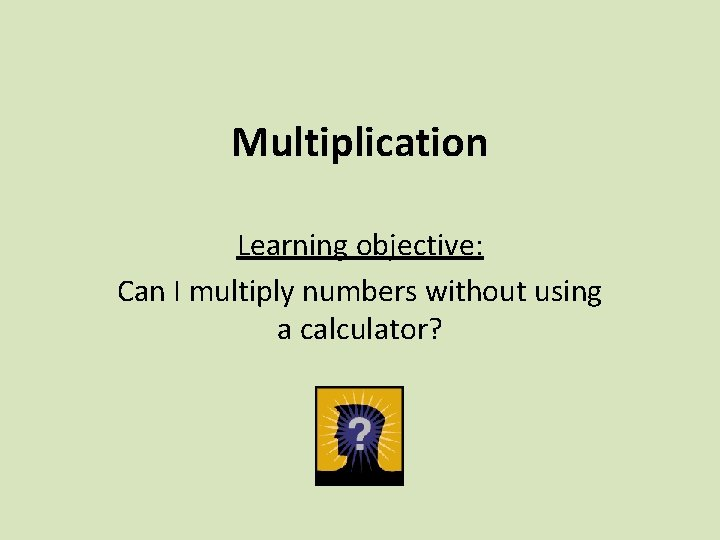Multiplication Learning objective: Can I multiply numbers without using a calculator?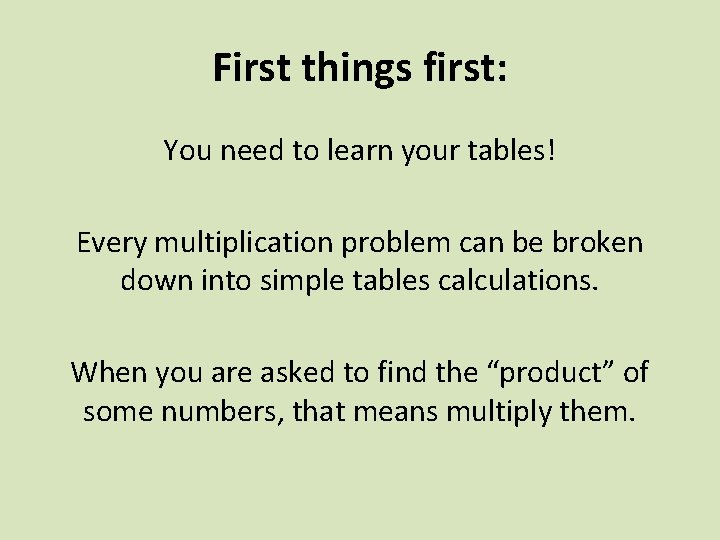First things first: You need to learn your tables! Every multiplication problem can be broken down into simple tables calculations. When you are asked to find the “product” of some numbers, that means multiply them.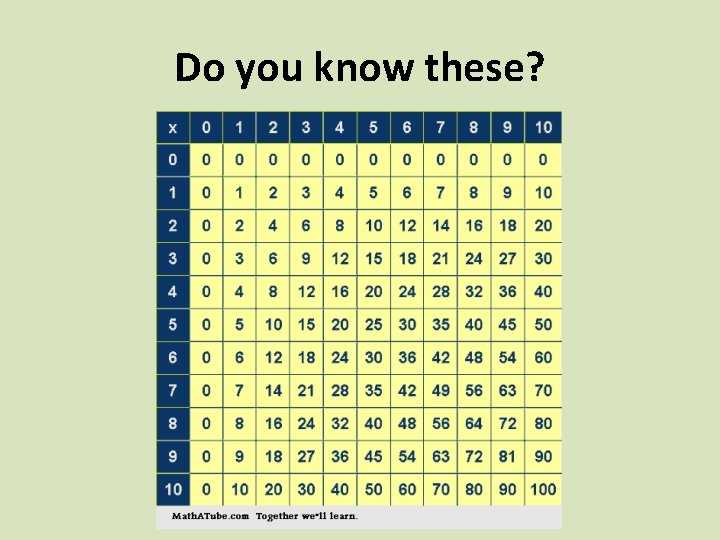Do you know these?Calculate 23 × 8 Split the large number up: This is 2 × 8 with a zero on the end 20 × 8 = 160 3 × 8 = 24 Add the answers together as you have a total of 23 eights. Answer: 184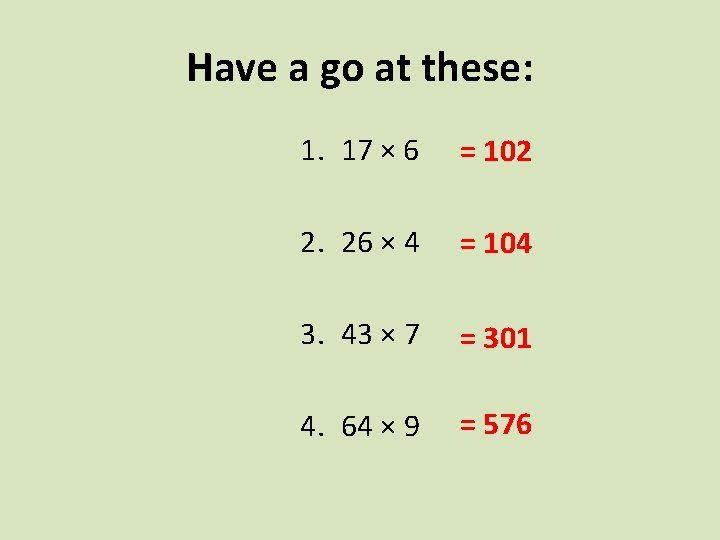Have a go at these: 1. 17 × 6 = 102 2. 26 × 4 = 104 3. 43 × 7 = 301 4. 64 × 9 = 576When they get a bit tougher: You have a few options. They all work just as well as each other. Choose the one that works for you.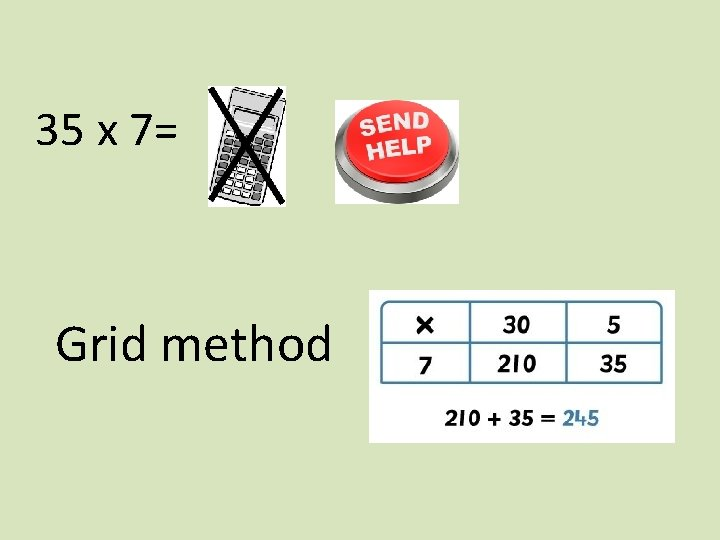35 x 7= Grid methodThe grid method can also be used for more difficult multiplications 30 5 x 35 x 26 20 600 100 6 180 30Have a go at calculating 21 × 32 Do it in as many ways possible…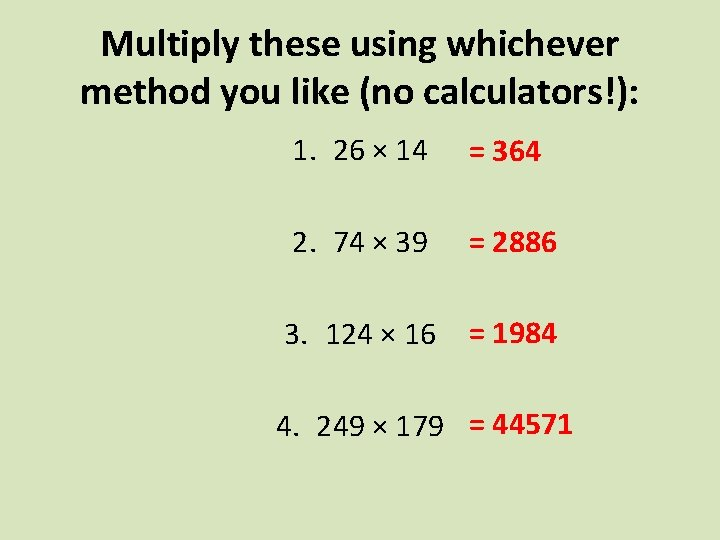Multiply these using whichever method you like (no calculators!): 1. 26 × 14 = 364 2. 74 × 39 = 2886 3. 124 × 16 = 1984 4. 249 × 179 = 44571Division Learning objective: Can I divide numbers without using a calculator?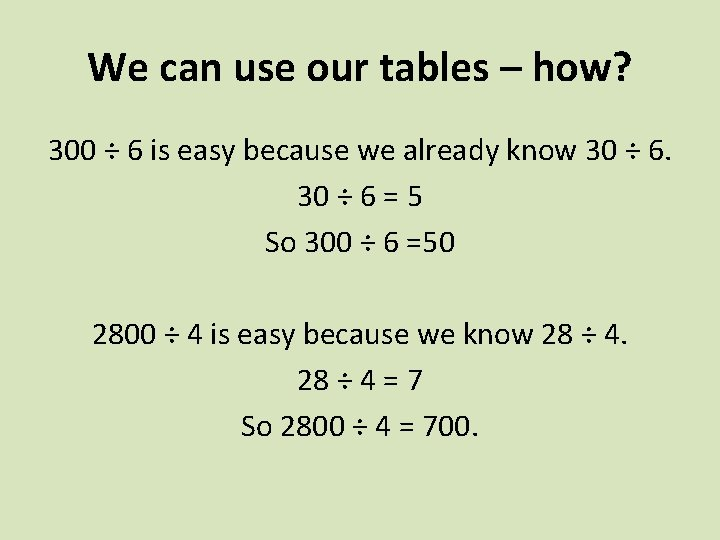We can use our tables – how? 300 ÷ 6 is easy because we already know 30 ÷ 6 = 5 So 300 ÷ 6 =50 2800 ÷ 4 is easy because we know 28 ÷ 4 = 7 So 2800 ÷ 4 = 700.Have a go at these: 1. 2000 ÷ 5 = 400 2. 320 ÷ 4 = 80 3. 33, 000 ÷ 3 = 11, 000 4. 42, 000 ÷ 7 = 6, 000Sometimes the division problems are a little tougher…The “bus stop” method You do need to know your tables! The most common method is called “the bus stop”, because it looks like the numbers are waiting in a bus stop. The divisor goes outside the “bus stop”. You can also add multiples of your divisor, but this will take you longer!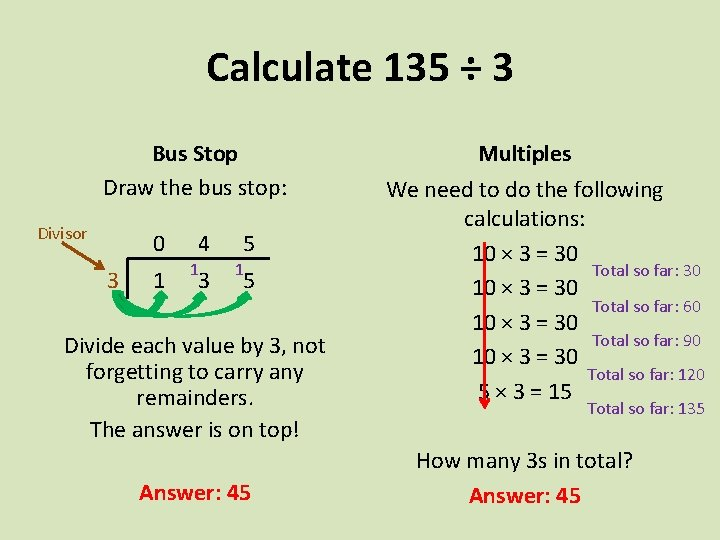Calculate 135 ÷ 3 Bus Stop Draw the bus stop: Divisor 0 3 1 4 1 3 5 1 5 Divide each value by 3, not forgetting to carry any remainders. The answer is on top! Answer: 45 Multiples We need to do the following calculations: 10 × 3 = 30 Total so far: 30 10 × 3 = 30 Total so far: 60 10 × 3 = 30 Total so far: 90 10 × 3 = 30 Total so far: 120 5 × 3 = 15 Total so far: 135 How many 3 s in total? Answer: 45What about 322 ÷ 14 Bus Stop Draw the bus stop: Divisor 0 14 3 2 3 4 2 Divide each value by 14, not forgetting to carry any remainders. The answer is on top! Answer: 23 Multiples We need to do the following calculations: 10 × 14 = 140 Total so far: 140 10 × 14 = 140 Total so far: 280 3 × 14 = 42 Total so far: 322 How many 14 s in total? Answer: 23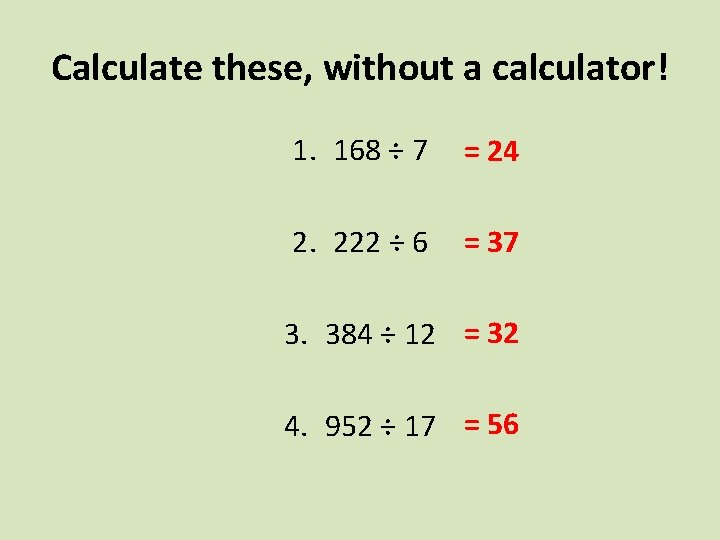Calculate these, without a calculator! 1. 168 ÷ 7 = 24 2. 222 ÷ 6 = 37 3. 384 ÷ 12 = 32 4. 952 ÷ 17 = 56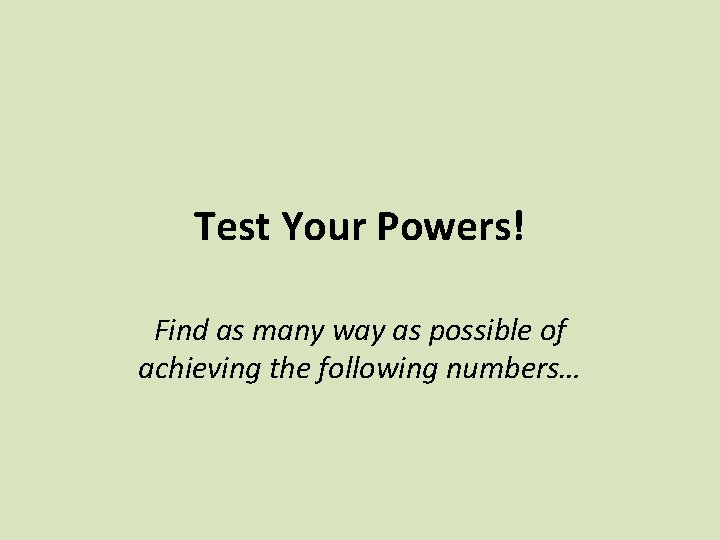Test Your Powers! Find as many way as possible of achieving the following numbers…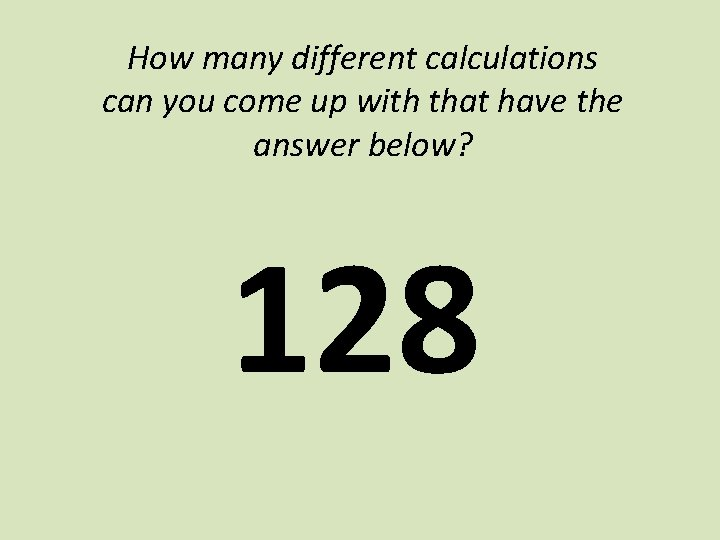How many different calculations can you come up with that have the answer below? 128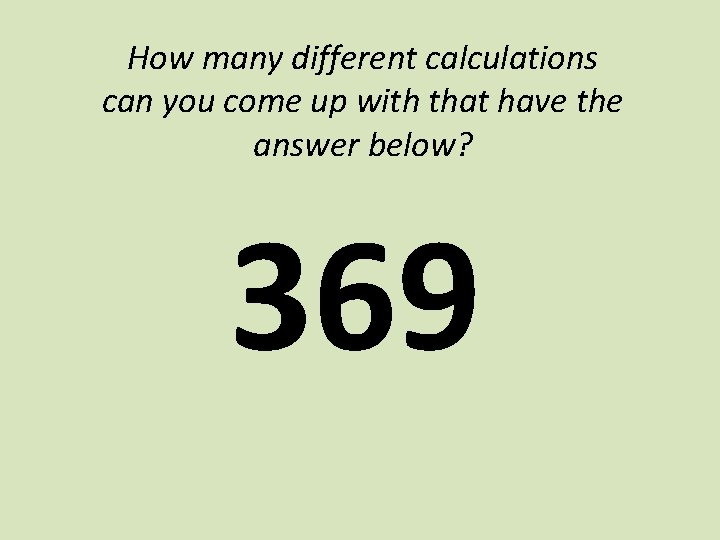How many different calculations can you come up with that have the answer below? 369How many different calculations can you come up with that have the answer below? 729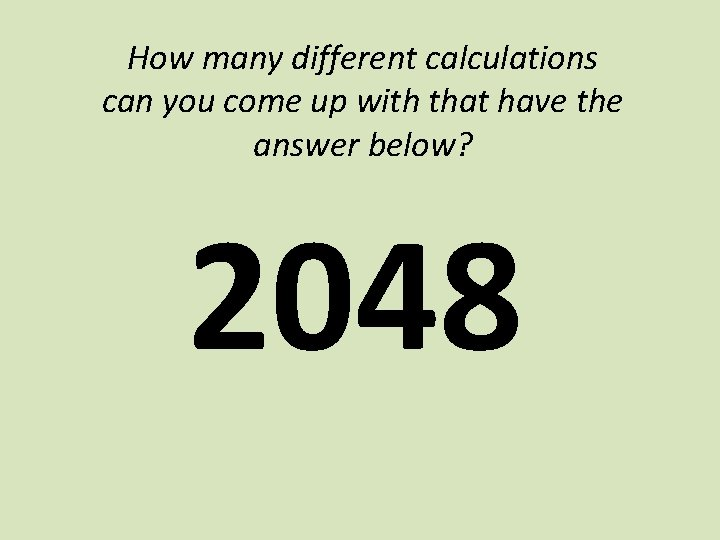How many different calculations can you come up with that have the answer below? 2048